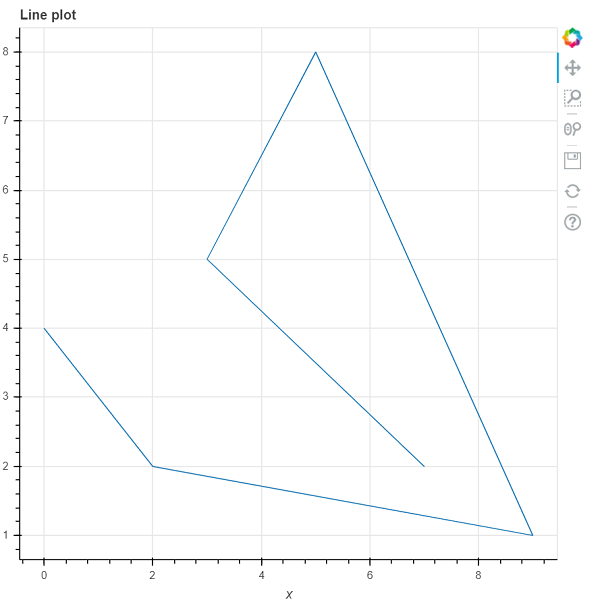# How can Bokeh library be used to generate line graphs in Python?

PythonServer Side ProgrammingProgramming

Bokeh is a Python package that helps in data visualization. It is an open source project. Bokeh renders its plot using HTML and JavaScript. This indicates that it is useful while working with web−based dashboards.

Visualizing data is an important steps since it helps understand what is going on in the data without actually looking at the numbers and performing complicated computations.

Bokeh can be easily used in conjunction with NumPy, Pandas, and other Python packages. It can be used to produce interactive plots, dashboards, and so on. It helps in communicating the quantitative insights to the audience effectively.

Bokeh converts the data source into a JSON file. This file is used as an input to BokehJS, which is a JavaScript library. This BokehJS is written in TypeScript that helps render visualization on modern browsers.

Matplotlib and Seaborn produce static plots, whereas Bokeh produces interactive plots. This means when the user interacts with these plots, they change accordingly.

Plots can be embedded as output of Flask or Django enabled web applications. Jupyter notebook can also be used to render these plots.

Dependencies of Bokeh −

Numpy
Pillow
Jinja2
Packaging
Pyyaml
Six
Python−dateutil

Installation of Bokeh on Windows command prompt

pip3 install bokeh


Installation of Bokeh on Anaconda prompt

conda install bokeh

Following is an example −

## Example

from bokeh.plotting import figure, output_file, show
x = [7, 3, 5, 9, 2, 0]
y = [2, 5, 8, 1, 2, 4]
output_file('sample.html')
fig = figure(title = 'Line plot ', x_axis_label = 'x', y_axis_label = 'y')
fig.line(x,y)
show(fig)

## Output## Explanation

• The required packages are imported, and aliased.

• The data is defined as two lists.

• The figure function is called.

• The ‘output_file’ function is called to mention the name of the html file that will be generated.

• The ‘line’ function present in Bokeh is called.

• The ‘show’ function is used to display the plot.